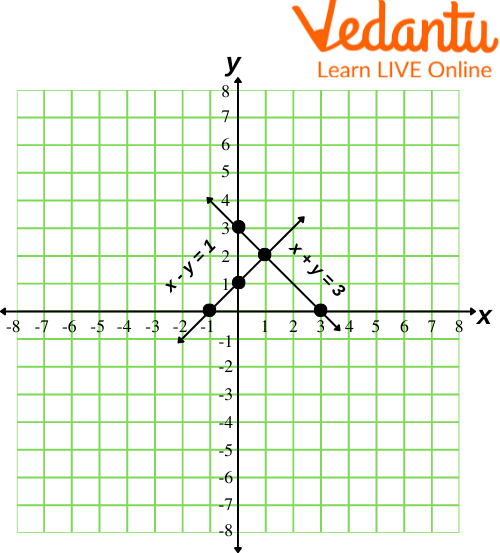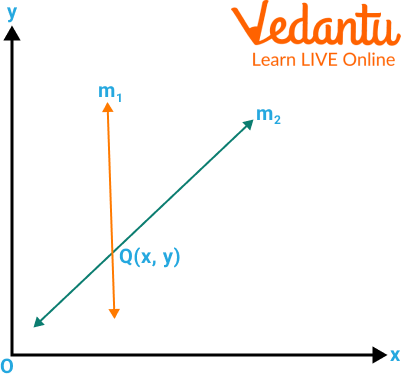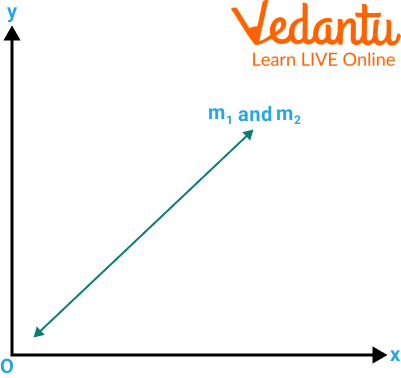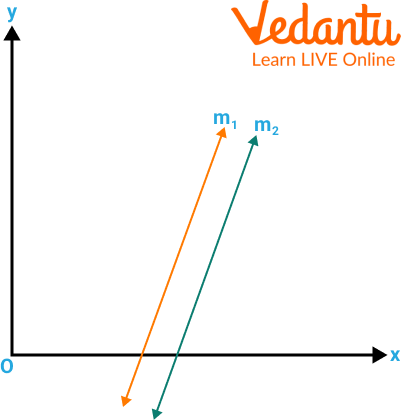Courses
Courses for Kids
Free study material
Free LIVE classes
More

# Solve Graphically - Linear EquationsLIVE
Join Vedantu’s FREE Mastercalss

## An Introduction to Linear Equations

The mathematical expressions of variables and constants along with the mathematical operations form an equation of the highest degree one. When plotted on a graph, a linear equation is an algebraic equation between variables that produces a straight line. A linear equation of one variable is of the form ax + b = 0 where x is the variable. Linear equations of two variables are of the form ax + by + c = 0 where x and y are the two variables and c is the constant. A pair of linear equations can be solved and represented using two basic methods: the graphical method and the algebraic method. In this article, we are going to learn about solving linear equations graphically.Examples of Linear Equations

## System of Linear Equations

A group of more than one linear equation is commonly known as a system of linear equations. For solutions, we can solve the system of linear equations graphically.

## Solving Linear Equations in Two Variables

The solution to linear equations comes in a pair (x, y), one value for the variable x and one value for the variable y. Thus, the solutions for any linear equation in two variables in the form ax+by-c = 0 represent a particular point on the cartesian plane. The graph of a linear equation is always a straight line, no matter what the solutions are.System of Linear Equations

## How to Plot the Equations on a Graph

• If there is one point of intersection, there is one solution. The x and y coordinates of the point of intersection are the values for the variables that are common between the two equations.One Solution

• If the lines coincide, then there are infinitely many solutions.Many Solution

• If the lines are parallel, there is no solution.No Solution

## Solved Example

1. Check whether the equation $y = 5x + 4$ is linear or not.

Ans: An equation is considered linear if it is in the form of $y=m x+b$ where $\mathrm{m}$ is the slope of the equation and $\mathrm{b}$ is the $\mathrm{y}$-intercept.

Here, $x$ can only be to the power of one.

Since $y = 5x + 4$ is in the form of $y=m x+b$ and x is having power 1. Therefore, the equation is linear.

2. Check whether the equation $y=3 x^2+1$ is linear or not.

Ans: Here, the power of x is more than 1. And for linearity, the power should be 1. Hence, the given equation is not linear.

3. What are the variable and contents in the given equation: $\dfrac{1}{\sqrt{2+x}}=1$

Ans:

• Variables: x

• Constants: 2

## Practice Questions

1. Check whether the equation $y = 9x + 10$ is linear or not.

Ans: Linear

2. Check whether the equation $y=2 x^5+10$ is linear or not.

Ans: Not Linear

3. Check whether the equation $y=4 x^2+ z$ is linear or not.

Ans: Not Linear

## Summary

A linear equation is in the form of ax+by+c=0, where a and b are not equal to zero. A linear equation in two variables is an equation that contains two variables (x, y) and the highest degree of the equation is 1. A group of more than one linear equation is known as a system of linear equations. The solution to a system of linear equations in two variables can be found by solving the system graphically. To solve it graphically, the equations have to be graphed individually and then their point(s) of intersection determines the common solution.

Last updated date: 22nd Sep 2023
Total views: 109.2k
Views today: 2.09k

## FAQs on Solve Graphically - Linear Equations

1. What is a system of Linear Equations and how can it be solved graphically?

A group of more than one linear equation is commonly known as a system of linear equations. To solve simultaneous equations graphically, we have to plot the points on a graph.

• One Solution - If the lines plotted for both the graphs only intersect at one point, then there exists only one common solution for the equations.

• No Solutions - If the lines plotted for the equations are parallel, i.e., do not intersect at any point, then the system of linear equations has no common solutions.

• Infinite Solutions - If the lines plotted for the system of equations coincide, then the two equations have infinitely many solutions.

In this way, we can solve the equations graphically and find the solutions.

2. Why are linear equations called linear equations?

Linear equations are called Linear Equations as the highest degree in the equation is one. A degree refers to the highest exponential power of a variable in the equation. In a linear equation, there is no exponential power (or it is 1) and thus its degree is considered to be 1. An equation with the highest power 2 is known as a quadratic equation. A linear equation can contain one variable, two variables, or even three of them.

3. Can a system of linear equations have zero solutions?

A system of linear equations can have zero, one, or an infinite number of solutions.